# dsp.SampleRateConverter

Multistage sample rate converter

## Description

The SampleRateConverter System object™ converts the sample rate of an incoming signal.

To convert the sample rate of a signal:

1. Create the dsp.SampleRateConverter object and set its properties.

2. Call the object with arguments, as if it were a function.

This object supports C/C++ code generation and SIMD code generation under certain conditions. For more information, see Code Generation.

## Creation

### Syntax

src = dsp.SampleRateConverter
src = dsp.SampleRateConverter(Name,Value)

### Description

src = dsp.SampleRateConverter creates a multistage FIR sample rate converter System object, src, that converts the sample rate of each channel of an input signal.

example

src = dsp.SampleRateConverter(Name,Value) returns a multistage FIR sample rate converter System object, src, with properties and options specified by one or more Name,Value pair arguments.

## Properties

expand all

Unless otherwise indicated, properties are nontunable, which means you cannot change their values after calling the object. Objects lock when you call them, and the release function unlocks them.

If a property is tunable, you can change its value at any time.

Specify the two-sided bandwidth of interest (after rate conversion) as a positive scalar expressed in Hz. This property sets the two-sided bandwidth of the information-carrying portion of the signal that you wish to retain.

Specify the sample rate of the input signal as a positive scalar expressed in Hz. The input sample rate must be greater than the bandwidth of interest.

Specify the maximum allowed tolerance for the sample rate of the output signal as a positive scalar between 0 and 1.

The output rate tolerance allows for a simpler design in many cases. The actual output sample rate varies but is within the specified range. For example, if OutputRateTolerance is specified as 0.01, then the actual output sample rate is in the range given by OutputSampleRate ± 1%.

Specify the sample rate of the output signal as a positive scalar expressed in Hz. The output sample rate must be greater than the bandwidth of interest.

Specify the stopband attenuation as a positive scalar expressed in dB. This property is the minimum amount by which any aliasing involved in the process is attenuated.

## Usage

### Syntax

y = src(x)

### Description

example

y = src(x) designs one or more multirate FIR filters and then uses the filters to convert the rate of each channel (column) of the real or complex input signal x to the output sampling rate.

### Input Arguments

expand all

Input signal, specified as a vector or a matrix of size P-by-Q. The number of input rows P can be arbitrary and does not have to be a multiple of the overall decimation factor. You can determine the decimation factor using the getRateChangeFactors function. The object treats each column of x as a separate channel.

This object supports variable-size input signals, that is, the frame length (number of rows) of the signal can change even when the object is locked. However, the number of channels (columns) must remain constant.

Data Types: single | double
Complex Number Support: Yes

### Output Arguments

expand all

Resampled signal, returned as a vector or matrix.

When the input is of size P-by-Q, the output signal has an upper bound size of ceil(P/R)-by-Q, where R is the ratio of the input sample rate and the output sample rate. The number of channels (columns) does not change.

Data Types: single | double
Complex Number Support: Yes

## Object Functions

To use an object function, specify the System object as the first input argument. For example, to release system resources of a System object named obj, use this syntax:

release(obj)

expand all

 getActualOutputRate Get actual output rate getFilters Obtain single-stage filters getRateChangeFactors Get overall interpolation and decimation factors visualizeFilterStages Visualize filter stages
 cost Implementation cost of the sample rate converter freqz Frequency response of the multirate multistage filter info Display information about sample rate converter
 step Run System object algorithm release Release resources and allow changes to System object property values and input characteristics reset Reset internal states of System object

## Examples

collapse all

Convert the sample rate of an audio signal from 44.1 kHz (CD quality) to 96 kHz (DVD quality).

Note: The dsp.AudioFileWriter System object™ is not supported in MATLAB Online.

FsIn = 44.1e3; FsOut = 96e3; SRC = dsp.SampleRateConverter('Bandwidth',40e3,... 'InputSampleRate',FsIn,'OutputSampleRate',FsOut); [L,M] = getRateChangeFactors(SRC); FrameSize = 10*M; AR = dsp.AudioFileReader('guitar10min.ogg', ... 'SamplesPerFrame',FrameSize); AW = dsp.AudioFileWriter('guitar10min_96k.wav', ... 'SampleRate',FsOut);

Convert 100 frames of audio signal. Release all objects.

for n = 1:100 x = AR(); y = SRC(x); AW(y); end release(AR); release(AW); release(SRC);

Plot the input and output signals. The latency of the sample rate converter introduces a delay in the output signal.

tx = (0:length(x)-1)./FsIn; ty = (0:length(y)-1)./FsOut; figure subplot(2,1,1) plot(tx,x(:,1),'.') hold on plot(ty,y(:,1),'--') ylim([-0.25 0.25]); xlim([0 0.005]) xlabel('Time (s)') legend('Input samples','Output samples','Location','best') title('Channel 1') subplot(2,1,2) plot(tx,x(:,2),'.') hold on plot(ty,y(:,2),'--') ylim([-0.25 0.25]); xlim([0 0.005]) xlabel('Time (s)') legend('Input samples','Output samples','Location','best') title('Channel 2')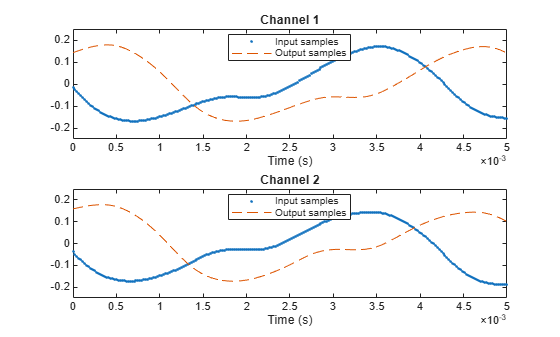Use the outputDelay function to determine this delay value. To account for this delay, shift the output by this delay value.

[delay,~,~] = outputDelay(SRC,Fc=0)
delay = 0.0012 
tx = (0:length(x)-1)./FsIn; ty = (0:length(y)-1)./FsOut; figure subplot(2,1,1) plot(tx,x(:,1),'.') hold on plot(ty-delay,y(:,1),'--') xlim([0 0.005]) xlabel('Time (s)') legend('Input samples','Output samples','Location','best') title('Channel 1') subplot(2,1,2) plot(tx,x(:,2),'.') hold on plot(ty-delay,y(:,2),'--') xlim([0 0.005]) xlabel('Time (s)') legend('Input samples','Output samples','Location','best') title('Channel 2')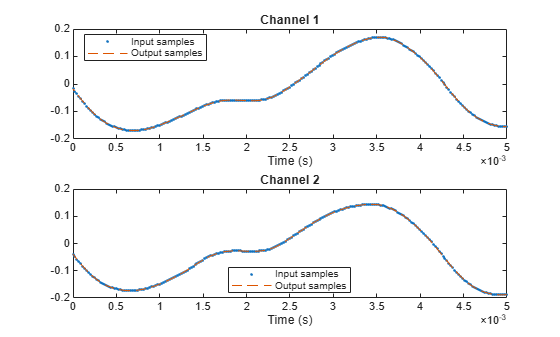Zoom in to see the difference in sample rates.

figure subplot(2,1,1) plot(tx,x(:,1),Color=[0.6 0.6 0.6]) hold on plot(tx,x(:,1),'ro') plot(ty-delay,y(:,1),'b.') xlim([0.0105 0.0107]) legend('Interpolated input','Input samples','Output samples') title('Channel 1') subplot(2,1,2) plot(tx,x(:,2),Color=[0.6 0.6 0.6]) hold on plot(tx,x(:,2),'ro') plot(ty-delay,y(:,2),'b.') xlim([0.0105 0.0107]) legend('Interpolated input','Input samples','Output samples') title('Channel 2')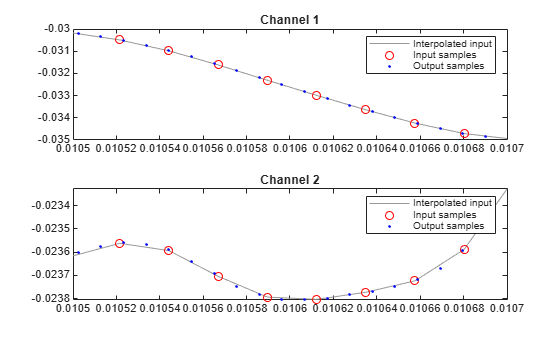Create a multistage sample rate converter with default properties. The converter converts from 192 kHz to 44.1 kHz in three stages.

src = dsp.SampleRateConverter;

Use src to convert the sample rate of a noisy sinusoid. The sinusoid has a frequency of 20 kHz and is sampled for 0.1 s.

f = 20e3; FsIn = src.InputSampleRate; FsOut = src.OutputSampleRate; t1 = (0:1/FsIn:0.1-1/FsIn)'; sIn = sin(2*pi*f*t1) + randn(size(t1));

Estimate the power spectral density of the input.

hsa = spectrumAnalyzer('SampleRate',FsIn,... 'Method','welch','YLimits',[-40 40]); hsa(sIn) release(hsa)Convert the sample rate of the signal. Estimate the power spectral density of the output.

sOut = src(sIn); hsb = spectrumAnalyzer('SampleRate',FsOut,... 'Method','welch','YLimits',[-40 40]); hsb(sOut) release(hsb)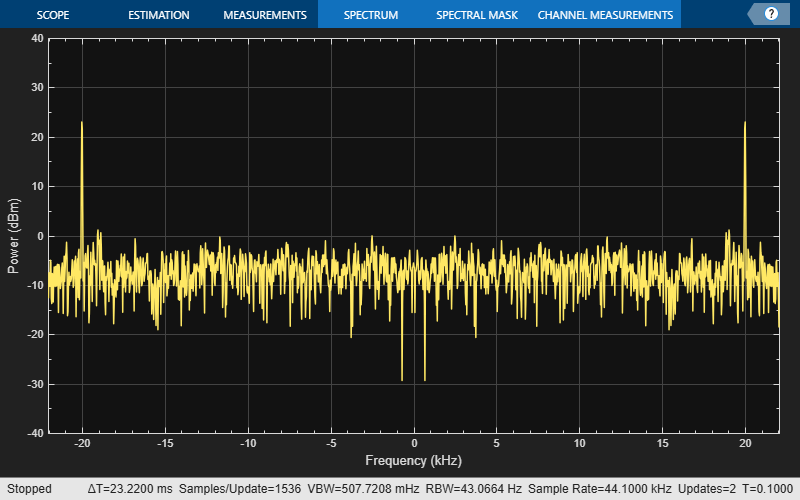A signal output from an A/D converter is sampled at 98.304 MHz. The signal has a bandwidth of 20 MHz. Reduce the sample rate of the signal to 22 MHz, which is the bandwidth of 802.11 channels. Make the conversion exactly and then redo it with an output rate tolerance of 1%.

SRC1 = dsp.SampleRateConverter('Bandwidth',20e6, ... 'InputSampleRate',98.304e6,'OutputSampleRate',22e6, ... 'OutputRateTolerance',0); SRC2 = dsp.SampleRateConverter('Bandwidth',20e6, ... 'InputSampleRate',98.304e6,'OutputSampleRate',22e6, ... 'OutputRateTolerance',0.01);

Use the cost method to determine the cost of each sample rate conversion. The zero-tolerance process requires more than 500 times as many coefficients as the 1% process.

c1 = cost(SRC1)
c1 = struct with fields: NumCoefficients: 84779 NumStates: 133 MultiplicationsPerInputSample: 27.0422 AdditionsPerInputSample: 26.0684 
c2 = cost(SRC2)
c2 = struct with fields: NumCoefficients: 150 NumStates: 127 MultiplicationsPerInputSample: 22.6667 AdditionsPerInputSample: 22.1111 

Find the integer upsampling and downsampling factors used in each conversion.

[L1,M1] = getRateChangeFactors(SRC1)
L1 = 1375 
M1 = 6144 
[L2,M2] = getRateChangeFactors(SRC2)
L2 = 2 
M2 = 9 

Compute the actual sample rate of the output signal when the sample rate conversion has a tolerance of 1%.

getActualOutputRate(SRC2)
ans = 2.1845e+07 

Create a multistage sample rate converter with default properties, corresponding to the combined three filter stages used to convert from 192 kHz to 44.1 kHz. Determine its overall decimation and interpolation factors.

src = dsp.SampleRateConverter; [L,M] = getRateChangeFactors(src);

Create a two-channel random signal. Specify a number of samples equal to the decimation factor. Call the object twice on the signal.

x = randn(M,2); y1 = src(x); y2 = src(x); no = all(y2==y1)
no = 1x2 logical array 0 0 

The output is different because the internal states of src have changed. Use reset to reset the converter and call the converter again. Verify that the output is unchanged.

reset(src) y3 = src(x); yes = all(y3==y1)
yes = 1x2 logical array 1 1 

Create a multistage sample rate converter with default properties, corresponding to the combined three filter stages used to convert from 192 kHz to 44.1 kHz. Compute and display the frequency response.

src = dsp.SampleRateConverter; [H,f] = freqz(src); plot(f,20*log10(abs(H)))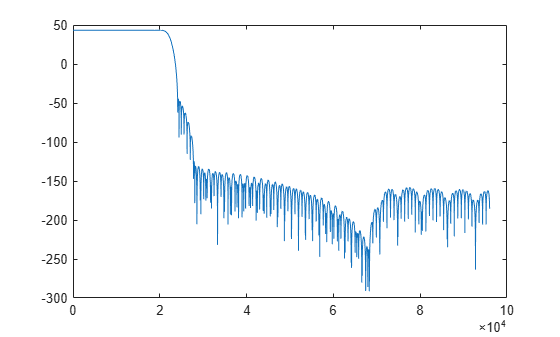Compute and display the frequency response over the range between 20 Hz and 44.1 kHz.

f = 20:10:44.1e3; [H,f] = freqz(src,f); plot(f,20*log10(abs(H)))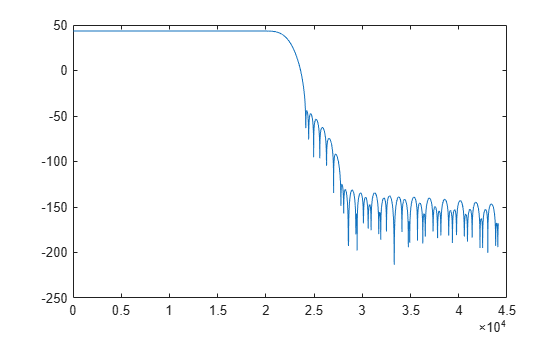Create src, a multistage sample rate converter with default properties. src converts between 192 kHz and 44.1 kHz. Find the individual filters that are cascaded together to perform the conversion.

src = dsp.SampleRateConverter; c = getFilters(src);

Visualize the frequency response of the decimator used in the first stage of the process.

m = c.Stage1; [h,w] = freqz(m); plot(w/pi,20*log10(abs(h))) xlabel('\omega / \pi') ylabel('Magnitude (dB)')Create a multistage sample rate converter with default properties, corresponding to the combined three filter stages used to convert from 192 kHz to 44.1 kHz.

src = dsp.SampleRateConverter
src = dsp.SampleRateConverter with properties: InputSampleRate: 192000 OutputSampleRate: 44100 OutputRateTolerance: 0 Bandwidth: 40000 StopbandAttenuation: 80 

info(src)
ans = 'Overall Interpolation Factor : 147 Overall Decimation Factor : 640 Number of Filters : 3 Multiplications per Input Sample: 27.667188 Number of Coefficients : 8631 Filters: Filter 1: dsp.FIRDecimator - Decimation Factor : 2 Filter 2: dsp.FIRDecimator - Decimation Factor : 2 Filter 3: dsp.FIRRateConverter - Interpolation Factor: 147 - Decimation Factor : 160 ' 

Get the actual output sample rate for conversion between 192 kHz and 44.1 kHz when given a tolerance of 1%.

src = dsp.SampleRateConverter; src.OutputRateTolerance = 0.01; FsOut = getActualOutputRate(src)
FsOut = 4.4308e+04 

Create src, a multistage sample rate converter with default properties. src combines three filter stages to convert from 192 kHz to 44.1 kHz. Determine its overall interpolation and decimation factors.

src = dsp.SampleRateConverter; [L,M] = getRateChangeFactors(src)
L = 147 
M = 640 

Create a multistage sample rate converter with default properties, corresponding to the combined three filter stages used to convert from 192 kHz to 44.1 kHz. Visualize the stages.

src = dsp.SampleRateConverter; visualizeFilterStages(src)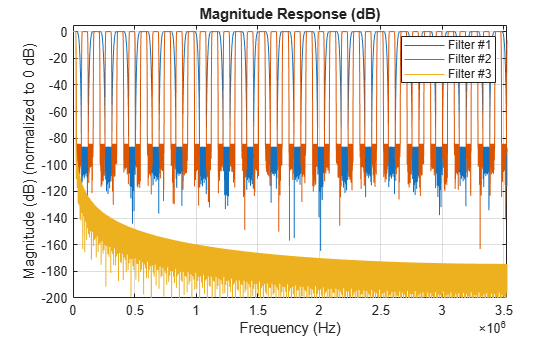## Algorithms

• The general multistage sample rate converter performs a multistage decimation, a single-stage sample rate conversion, and a multistage interpolation. Actual designs include at most two of these steps.

• The procedure automatically determines the optimal number of decimation or interpolation stages. In special cases, the algorithm can perform a decimation or interpolation in a single stage.

• The algorithm always attempts to start by reducing the sample rate. This decreases the amount of computation required. The decimation step ensures that the intermediate sample rate does not go below the bandwidth of interest and that the block does not filter out any information.

• Each individual stage uses halfband or Nyquist filters to minimize the number of nonzero coefficients.

• The algorithm allows transition-band aliasing because it decreases the implementation cost. It keeps the signal within the bandwidth of interest alias free up to the value specified by the StopbandAttenuation property.

## Version History

Introduced in R2014b

expand all